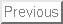M403K First Midterm Exam Solutions.  September 21, 2000

1. Consider the function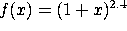a) Find f'(x).

Soln: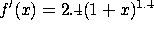b) Find the slope of the line tangent to the curve y=f(x) at x=0.

Soln: Slope equals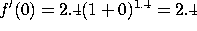c) Find the equation of this tangent line.

Soln: Since f(0)=1, the tangent line is y-1 = 2.4(x-0), or y = 2.4 x + 1.

d) Use this tangent line to approximate f(0.01).

Soln: So f(0.01) is very close to 2.4(0.01) + 1 = 1.024.

2. From the following table, estimate f'(1). Indicate clearly how you obtain your answer: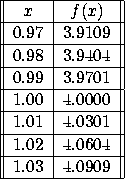Solution: For h ranging from -.03 to +0.03, look at [f(1+h)-f(1)]/h: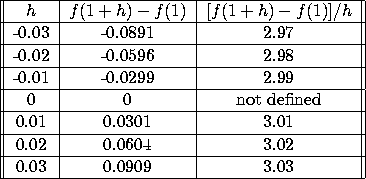As h approaches zero from either side, [f(1+h)-f(1)]/h approaches 3, so f'(1) equals 3.

3. Evaluate the following limits, if they exist (or write DNE if they do not).

a)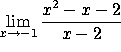As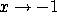, the numerator goes to zero while the denominator goes to -3, so the ratio goes to zero.

b)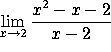As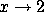both the numerator and denominator go to zero, so we have to be more careful. The numerator equals (x-2)(x+1). Canceling a factor of (x-2) from numerator and denominator we have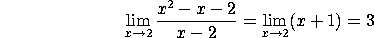c)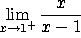As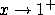, the numerator goes to 1 while the denominator goes to zero from above, so the limit is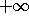. Put another way, if x is slightly bigger than 1 (say 1.001), then the numerator is close to 1 while the denominator is a small possitive number (like .001), so the ratio is a large positive number (like 1000).

d)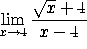As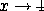, the numerator goes to 6, but the denominator goes to zero. If x is slightly bigger than 4, then the denominator is a small positive number and the ratio is a large positive number. That is,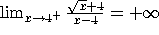. But if x is slightly less than 4, then the denominator is a small negative number and the ratio is a large negative number. That is,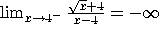. Since the limits from the two sides do not agree, there is no overall limit. The correct answer is ``DNE''.

4. Take the derivatives of the following functions with respect to x. You do not need to simplify your answers:

a)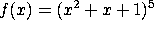Use the chain rule: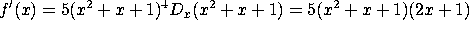.

b)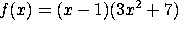Use the product rule: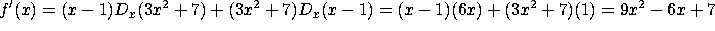.

c)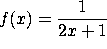You can either use the quotient rule or the chain rule. Since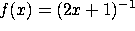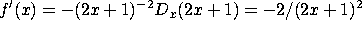.

d)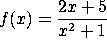Use the quotient rule.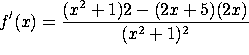.

5. New England Widget Technologies (NEWT) makes expensive high-tech widgets. Their marketing department has determined that the demand function is x = 4000 - 2p, where x is the number of widgets sold and p is the price. Their cost function is C(x)= 200,000 + 1000x.

a) Find the price p(x) and the revenue R(x) as a function of x.

Soln: p(x) = (4000-x)/2 = 2000 - x/2.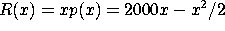.

b) Compute the marginal cost, marginal revenue and marginal profit as a function of x.

Soln: Marginal cost = C'(x) = 1000. Marginal revenue = R'(x)=2000-x. Marginal profit = P'(x) = R'(x)-C'(x) = 1000 - x.

c) The company has a current production level of x=1500. To increase revenue, should the company increase or decrease production? [Note: you do not need to compute the optimal level of production. You just need to say whether it is higher or lower than 1500.]

Soln: Since R'(1500)=2000-1500=500, each additional widget produced will increase revenue by \$500. So to increase revenue the company should increase production.

c) The company has a current production level of x=1500. To increase profit, should the company increase or decrease production?

Soln: Since P'(1500)=1000-1500=-500, each additional widget made will decrease profits by \$500. This makes sense, because each additional widget costs \$1000 to make and only brings in \$500 in revenue. So, to increase profit, the company should decrease production.